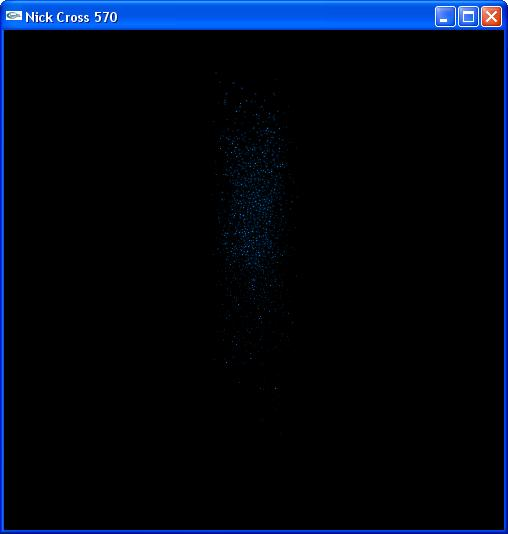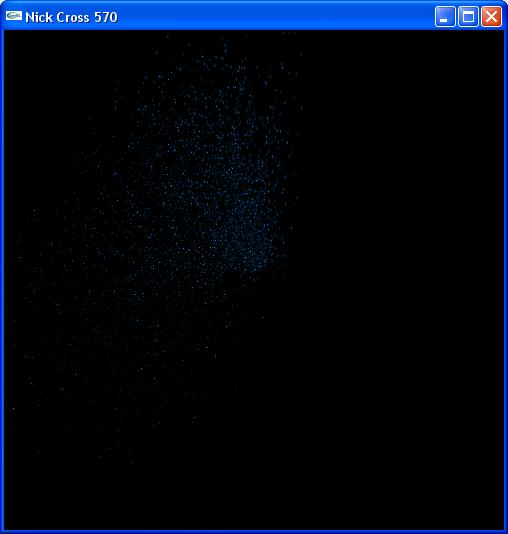Nick Cross - 570R Final Project

Winter '06Abstract:

I present a particle system where the all of the acceleration, color, and position vectors are calculated in a vertex program on the graphics processing unit (GPU) using C for Graphics (Cg). All vertices are drawn using the OpenGL on the central processing unit (CPU). The particle system starts particles from rest and shoots them into the air at random velocities. The particles then are controlled by gravity and a wind force completely independent of the CPU. The goal is to have a particle system that runs faster, more smoothly, and had a more simple approach.

Background:

Cg is a GPU programming language that can be run with many different programming languages. I choose to use C++ and OpenGL to interact with it. There are two different types of programs for the GPU: vertex, and fragment programs. Cg uses vectors in an accelerated way, so programming and manipulating vectors with Cg is recommended and necessary.

Vertex programs are Cg programs that calculate new positions, colors, lighting effects, and other similar computations on a per-vertex basis. This means that in the graphic pipeline receives a particle that was sent by OpenGL, it will use the position, texture, and any specified parameters to perform any calculations done inside the vertex program. Out variables are defined inside vertex programs which represent the data that will continue down the pipeline. All the information besides the new position vector will be used in the running fragment program.

Fragment programs, or pixel shaders, take in the parameters that were returned by a vertex program and specified parameters that have values assigned into them. It returns only a single color, which is the new pixel color. These are used for sampling textures and applying them to the current diffusalmap.

Results:

I present a particle system that only designates GL_QUADS to be drawn centered around the origin with sides .2 long. There is a wind force assigned, which can be be changed via the keyboard (see the table below). There is a global time step, which increments time for the GPU's position calculation. Right before the vertices are drawn, the initial position, velocity, force, mass, current time step, and current model view matrix of that particle are passed into the vertex program. The coordinates have an image texture mapped to so it's not just pixels moving on the screen.

The vertex program takes the inputed force and divides it by the mass to get an acceleration. It then adds the gravity to the acceleration. That acceleration is used in a simple position calculation that uses the initial position, velocity, and given time step to compute a new position. A turquoise color is assigned to the ouput color, and a point size based on the mass is assigned.

 // Based of particle.cg from CG tutorial void main( float4 pInitial : POSITION, float4 myTex : TEXCOORD0, float tInitial : TEXCOORD1, float4 vInitial : TEXCOORD2, float4 force : TEXCOORD3, float mass : TEXCOORD4, out float4 oPosition : POSITION, out float4 oMyTex : TEXCOORD0, out float4 color : COLOR, out float pointSize : PSIZE, uniform float globalTime, uniform float4 gravity, uniform float4x4 modelViewProj) { float t = globalTime - tInitial; float4 acceleration = gravity + (force / mass); float4 pFinal = pInitial + vInitial * t + .5 * acceleration * t * t; oPosition = mul(modelViewProj, pFinal); color = float4(0,.5,1,1); oMyTex = myTex; pointSize = mass; }

Text 1 - particle.cg

The fragment program takes the out color, which is the texture defined for each vertex, and multiplies it by the current diffuse map. This samples the texture for that specific pixel. I then multiply that result by the out color, which shades the pixel a turquoise color.

 // Modified particle_texture.cg from cg tutorial void frag_particle(float2 texCoord : TEXCOORD0, float4 color : COLOR, out float4 oColor : COLOR, uniform sampler2D diffuseMap) { oColor = tex2D(diffuseMap,texCoord) * color; }

Text 2- particleFrag.cg

The system works fast and appears to be of higher quality and speed than a CPU driven particle system.

Screenshots:Startup viewWind force to the left.

Commands:

Key

Result

a

Wind +x

d

Wind -x

w

Wind +y

d

Wind -y

e

Wind +z

q

Wind -z

References and Resources:

Fernando, Randima. Cg: The Cg Tutorial: The Definitive Guide ot Programmable Real-Time Graphics. 2003. Addison-Wesley. Boston.

final.zip